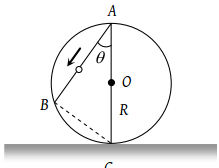A body is released from a great height and falls freely towards the earth. Another body is released from the same height exactly one second later. The separation between the two bodies, two seconds after the release of the second body is

(1) 4.9 m

(2) 9.8 m

(3) 19.6 m

(4) 24.5 m

Concept Questions :-

Uniformly accelerated motion
High Yielding Test Series + Question Bank - NEET 2020

Difficulty Level:

An object is projected upwards with a velocity of 100 m/s. It will strike the ground after (approximately)

(1) 10 sec

(2) 20 sec

(3) 15 sec

(4) 5 sec

Concept Questions :-

Uniformly accelerated motion
High Yielding Test Series + Question Bank - NEET 2020

Difficulty Level:

A stone dropped from the top of the tower touches the ground in 4 sec. The height of the tower is about

(1) 80 m

(2) 40 m

(3) 20 m

(4) 160 m

Concept Questions :-

Uniformly accelerated motion
High Yielding Test Series + Question Bank - NEET 2020

Difficulty Level:

A body is released from the top of a tower of height h. It takes t sec to reach the ground. Where will be the ball after time t/2 sec

(1) At h/2 from the ground

(2) At h/4 from the ground

(3) Depends upon mass and volume of the body

(4) At 3h/4 from the ground

Concept Questions :-

Uniformly accelerated motion
High Yielding Test Series + Question Bank - NEET 2020

Difficulty Level:

A frictionless wire AB is fixed on a sphere of radius R. A very small spherical ball slips on this wire. The time taken by this ball to slip from A to B is(1) $\frac{2\sqrt{gR}}{g\mathrm{cos}\theta }$

(2) $2\sqrt{gR}.\frac{\mathrm{cos}\theta }{g}$

(3) $2\sqrt{\frac{R}{g}}$

(4) $\frac{gR}{\sqrt{g\mathrm{cos}\theta }}$

Concept Questions :-

Uniformly accelerated motion
High Yielding Test Series + Question Bank - NEET 2020

Difficulty Level:

A body is slipping from an inclined plane of height h and length l. If the angle of inclination is θ, the time taken by the body to come from the top to the bottom of this inclined plane is

(1) $\sqrt{\frac{2h}{g}}$

(2) $\sqrt{\frac{2l}{g}}$

(3) $\frac{1}{\mathrm{sin}\theta }\sqrt{\frac{2h}{g}}$

(4) $\mathrm{sin}\theta \sqrt{\frac{2h}{g}}$

Concept Questions :-

Uniformly accelerated motion
High Yielding Test Series + Question Bank - NEET 2020

Difficulty Level:

A particle is projected up with an initial velocity of $80ft/\mathrm{sec}$. The ball will be at a height of $96ft$ from the ground after (Given  )

(1) 2.0 and 3.0 sec

(2) Only at 3.0 sec

(3) Only at 2.0 sec

(4) After 1 and 2 sec

Concept Questions :-

Uniformly accelerated motion
High Yielding Test Series + Question Bank - NEET 2020

Difficulty Level:

A body falls from rest, its velocity at the end of first second is $\left(g=32ft/{\mathrm{sec}}^{2}\right)$

(1) $16\text{\hspace{0.17em}\hspace{0.17em}}ft/\mathrm{sec}$

(2) $\text{32\hspace{0.17em}\hspace{0.17em}}ft/\mathrm{sec}$

(3) $64\text{\hspace{0.17em}\hspace{0.17em}}ft/\mathrm{sec}$

(4) $24\text{\hspace{0.17em}\hspace{0.17em}}ft/\mathrm{sec}$

Concept Questions :-

Uniformly accelerated motion
High Yielding Test Series + Question Bank - NEET 2020

Difficulty Level:

A stone thrown upward with a speed u from the top of the tower reaches the ground with a velocity 3u. The height of the tower is

(1) $3{u}^{2}/g$

(2) $4{u}^{2}/g$

(3) $6{u}^{2}/g$

(4) $9{u}^{2}/g$

Concept Questions :-

Uniformly accelerated motion
High Yielding Test Series + Question Bank - NEET 2020

Difficulty Level:

Two stones of different masses are dropped simultaneously from the top of a building

(1) Smaller stone hit the ground earlier

(2) Larger stone hit the ground earlier

(3) Both stones reach the ground simultaneously

(4) Which of the stones reach the ground earlier depends on the composition of the stone

Concept Questions :-

Uniformly accelerated motion